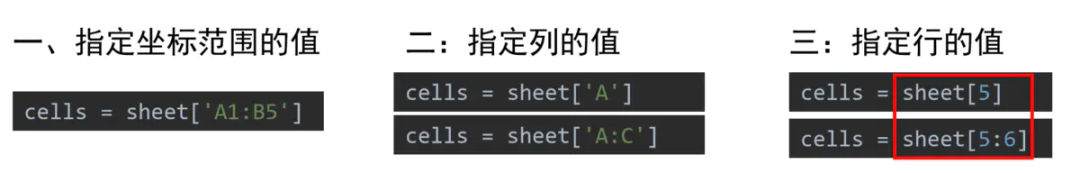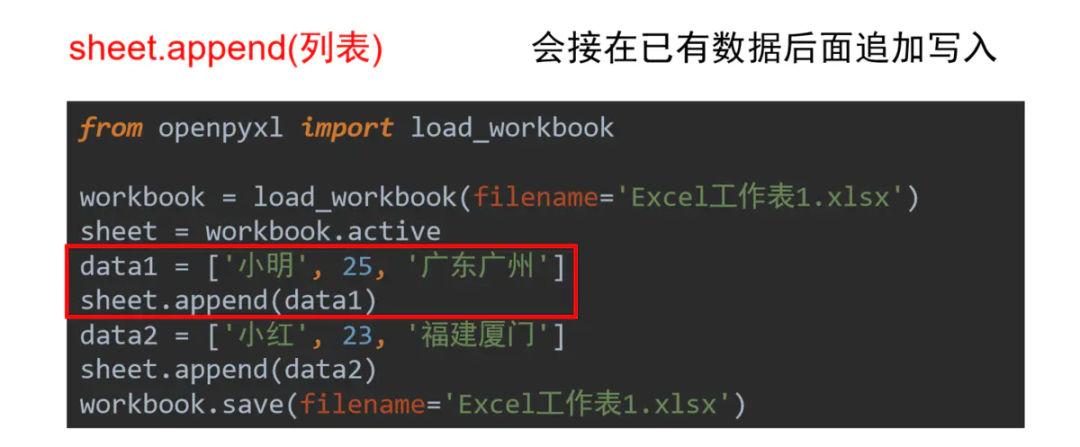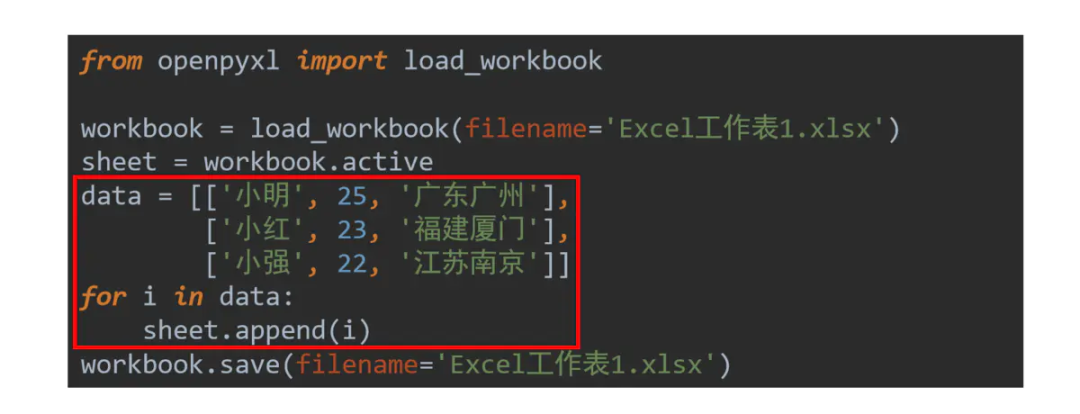# 多图+代码 | 详解Python操作Excel神器openpyxl的各种操作！【python自动化

## 安装

`openpyxl`是一个非标准库，因此需要自行安装，安装过程并不困难，Windows/Mac用户均可以在命令行(CMD)/终端(Terminal)中使用pip安装

``````pip install openpyxl
``````

## 读取Excel

### 1.载入Excel

``````from openpyxl import load_workbook
workbook = load_workbook(filename='测试.xlsx’)
print(workbook.sheetnames)
``````

### 2.根据名称获取工作表

``````from openpyxl import load_workbook
workbook = load_workbook(filename='其他.xlsx')
print(workbook.sheetnames)
sheet = workbook['工作业务']
``````

``````sheet = workbook.active
``````

### 3.获取表格内容所在的范围

``````print(sheet.dimensions)
``````

### 5.获取某个单元格的行、列、坐标

``````print(cell.row, cell.column, cell.coordinate)
``````

### 6.获取多个格子的值这里也有一个细节，Excel中每一列由字母确定，是字符型；每一行由一个数字确定，是整型。当然，上面的三种方法都是获取一堆表格，现在要输出每一个表格的值就需要遍历

``````for cell in cells:
print(cell.value)
``````

### 7.读取所有的行

``````for row in sheet.rows:
print(row)
``````

## Excel写入

### 1. 保存Excel

``````workbook.save(filename='Excel工作表1.xlsx')
``````
• 如果读取和写入Excel的路径相同则为对原文件进行修改
• 如果读取和写入Excel的路径不同则为保存成新的文件

### 2.写入单元格

``````cell = sheet['A1']
cell.value = '业务需求
``````

### 3.写入一行或多行数据### 4.将公式写入单元格并保存

``````sheet['K11'] = '=AVERAGE(K1:K10)'
``````

### 10. 创建新的Excel表格

``````from openpyxl import Workbook
workbook = Workbook()
``````

## Excel样式调整

### 2. 设置对齐样式

• 水平对齐:`distributed, justify, center, left, fill, centerContinuous, right, general`
• 垂直对齐:`bottom, distributed, justify, center, top`

### 3. 设置边框样式

• 边线样式:`double, mediumDashDotDot, slantDashDot, dashDotDot, dotted, hair, mediumDashed, dashed, dashDot, thin, mediumDashDot, medium, thick`

### 5. 设置行高和列宽

``````sheet.row_dimensions.height = 50
sheet.column_dimensions['C'].width = 20
``````

### 6. 单元格合并与取消

``````# 合并
sheet.merge_cells('A1:B2')
sheet.merge_cells(start_row=1, start_column=3,
end_row=2, end_column=4)

# 取消合并
sheet.unmerge_cells('A1:B2')
sheet.unmerge_cells(start_row=1, start_column=3,
end_row=2, end_column=4)``````